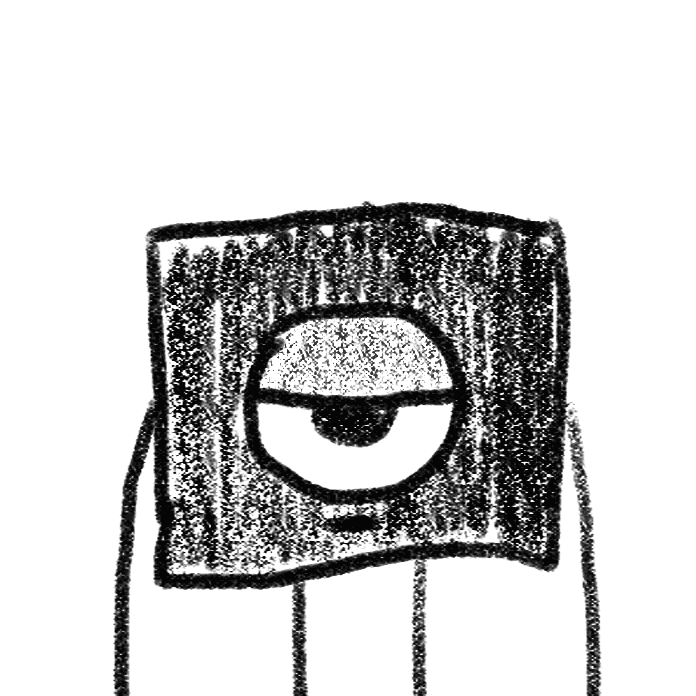# xⁿ + yⁿ = 1

Best definition
xÃ¢ÂÂ¿ + yÃ¢ÂÂ¿ = 1
The equation of a square as n tends to infinity, compared to the equation of a circle when n is

equal to two.

Substituting different values of n into the equation xÃ¢ÂÂ¿ + yÃ¢ÂÂ¿ = 1, using a computer software, is a pretty addictive activity even among the mathematically challenged and the retired.via giphy